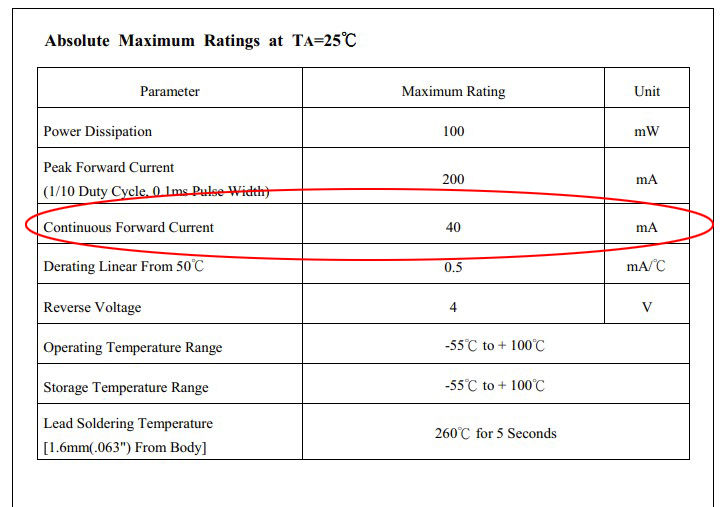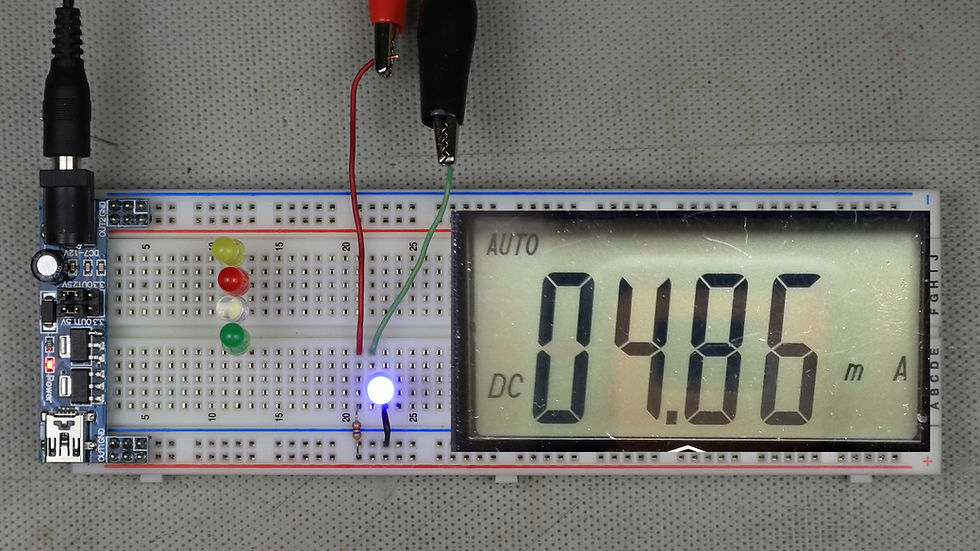top of page
Search

# LED BASICS AND HOW TO USE THEMFrom simple discrete indicators to large, high intensity lighting, the LED is everywhere these days.

After reading this article you will be confident in the basic usage of the humble LED and understand the most important characteristics when designing with them.

### HISTORY

The LED or Light Emitting Diode was first demonstrated in 1927 by Russian inventor Oleg Losev, however practical use of the LED wasn't realized until 1961 with the invention of the Near-Infrared (NIR) LED by James R. Biard and Gary Pittman at Texas Instruments.

The first visible LED, red in color, was demonstrated by Nick Holonyak, Jr. in 1962 at General Electric. Blue LED's came on the scene in 1972 at Stanford University by students Herb Maruska and Wally Rhines.

*History summarized from Wikipedia.

### OPERATION

The LED operates by passing a current through a semiconducting diode that has been doped with various elements such as Indium, Gallium, Aluminum, Phosphate, among others in order to emit photons of a certain wavelength. The voltage necessary for the LED to emit light increases as the wavelength shortens towards blue as we will see later.

### BASIC CIRCUITSimple LED circuit

This is the most basic circuit to drive an LED and consists of a voltage source, in this case being 5v, a resistor (470 ohms) and an LED in series. The resistor in series is important because LED's are current controlled devices meaning that the brightness is determined by the amount of current flowing through them. Low current, low brightness, high current, high brightness.

The other interesting feature is that being a diode, the voltage is constant across it (Vf) independent of the current through it (If) because of the depletion layer at the PN junction in the LED. This voltage becomes important when designing the LED circuit.

### IMPORTANT CHARACTERISTICS

LED data sheets contain many different parameters but the 2 most important when designing with them is the forward voltage (Vf) and the continuous forward current (If). There are others that may be important in certain applications such as the maximum reverse voltage and power dissipation. But for this basic understanding, we will concern ourselves with forward voltage and forward current.LED datasheet showing forward voltageThe 2 images above are from a typical LED datasheet with the forward voltage and forward current circled. So what do these mean?

### FORWARD VOLTAGE

The forward voltage is the voltage measured across the LED when lit, in this case it is typically 1.8v when there is 20mA of current passing through the LED. You will notice however that it also specifies a max voltage meaning that due to variations in manufacturing, the forward voltage can vary but is guaranteed to not exceed the value of 2.4v

So why is this important? Let's say you have a circuit that has a voltage of 2v. Most of the LED's you use will be at 1.8v which is fine because it is less than the 2v of our circuit. But what if you happen to pick one that is at 2.4v? When you power the circuit, the voltage will be less than the forward voltage and the LED will not illuminate. This can be an important consideration in low voltage situations. Since a lot of hobby electronics run at 3.3v or 5v, generally speaking there shouldn't be any reason to be concerned with the max Vf.

### FORWARD CURRENT

The continuous forward current is the maximum current that the LED can handle without damage, in this case it's 40mA. It's important to note that there is a peak forward current and this is the current that the LED can handle for brief periods. Typically this is rated for 10% duty cycle with 0.1mS maximum on time. Meaning you can drive the LED at 1KHz with it being on for 0.1mS and off for 0.9mS safely. For this discussion though, we will only consider it to be always on and use the continuous forward current only.

### CALCULATIONS

Now that we have the main characteristics, it is possible to design our LED circuit.Looking at the circuit again we see that there is a current limiting resistor in series with the LED. Using Kirchoff's Voltage Law (KVL), the sum of the voltage drops across the components must add up to the applied voltage.

This means that the voltage across the LED plus the voltage across the resistor must add up to the battery voltage.

Vt = Vr + Vf

Using the 1.8v from the data sheet and the 5v from the battery we get:

5v = Vr + 1.8v

Next we solve for Vr:

Vr = 5v - 1.8v

= 3.2v

Knowing Vr, we can calculate the current through the LED (If) using Ohm's Law:

V = I x R

3.2v = If x 470 ohms

Rearranging for If:

If = 3.2v / 470 ohms

= 6.8mA

In this example circuit, the current is 6.8mA, but what if we want to run the LED continuously at the brightest possible? Then you need to work backwards. Regardless if the current is 6.8mA or 40mA, the forward voltage will be the same so we can simply rearrange Ohms law and solve for R:

R = 3.2v / 40mA

= 80 ohms

Replacing the 470 ohm resistor with an 80 ohm resistor will give maximum brightness safely.

What happens if the LED forward voltage happens to be at the maximum? Recalculating Vr and the forward current gives:

Vr = 5v - 2.4v = 2.6v

If = 2.6v / 80 ohms = 32.5mA

Since If is less than the continuous forward current, we don't have to worry about LED variations as long as we calculate the current limiting resistor using the typical forward voltage as the current will drop with an increase in the forward voltage.

### EXAMPLE

Below are images of the example circuit from above with the actual measurements for Vf, Vr, and If. This particular LED is blue and has a larger Vf.Voltage across the LED. Forward Voltage (Vf)Voltage across the Resistor (Vr)Current through LED. Continuous Forward Current (If)

Doing the calculations for If:

If = 2.281v / 470 ohms = 4.85mA

Almost exactly as measured. And if we add the 2 voltages, Vr and Vf:

Vt = 2.281v + 2.730v = 5.011v

Also almost exactly as expected. (the 5v source was slightly greater than 5v)

### COLOR VS FORWARD VOLTAGE

Below is a collection of images of 5 different LED's and their measured forward voltages. As stated earlier, as the wavelength shortens, the forward voltage increases. From these images you see that red has the lowest Vf since it has the longest wavelength and the blue led has the highest Vf and the shortest wavelength. It's interesting to note that the white LED has the highest Vf as it uses phosphors to modify the color with a blue LED leading to a higher Vf.Red LED forward VoltageYellow LED Forward VoltageGreen LED Forward VoltageBlue LED Forward VoltageWhite LED Forward Voltage

### SUMMARY

LED's are everywhere. They are cheap, plentiful and come in a variety of colors. Perfect for the hobbiest to add to any project whether for aesthetics or to serve a useful purpose. LED's are easy to use and only the current limiting resistor needs to be calculated in most instances and can be done in 3 steps:

1. Determine Forward Voltage (Vf) of the LED.

2. Decide on the Forward Current up to the Continuous Forward Current (If).

3. Calculate the current limiting resistor:

R = (Applied Voltage - Vf ) / I

Now make something bright!